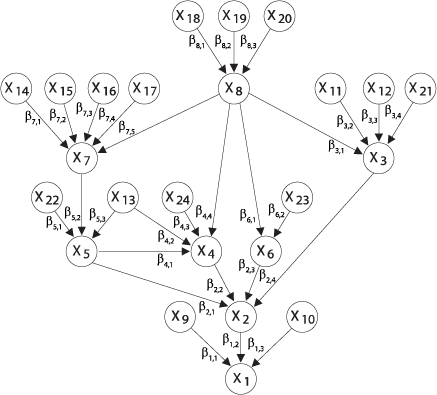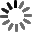El sitio web de la UCLM utiliza cookies propias y de terceros con fines técnicos y de análisis, pero no recaba ni cede datos de carácter personal de los usuarios. Sin embargo, puede haber enlaces a sitios web de terceros, con políticas de cookies distintas a la de la UCLM, que usted podrá aceptar o no cuando acceda a ellos.

Puede obtener más información en la Política de cookies. Aceptar# Mathematical model building

Curso de fuentes OpenUCLM

This course discusses how to build mathematical models to reproduce the physical and engineering reality. First, the Buckingham theorem is given together with some examples to illustrate the importance of using dimensionless ratios or variables, in order to reduce complexity and to avoid dimensional problems. We also discuss the concepts of complete and incomplete self-similarity, which plays a relevant role in model building. The concept of consistency of models is introduced and described. It includes dimension, physical, statistical and extreme value consistencies, which are analyzed in detail. For the sake of illustration, we also discuss multivariate models and provide very interesting examples of fatigue models, including the S-N curves, the crack growth curves and how they can be connected.

Autores Fecha Enrique Castillo 17/09/2019 Ingles

Me GustaIn this part the course is motivated. It is indicated how incorrect models appear in some books and research articles, which produce an important damage to society. This fact is illustrated with five examples that provide a first look to the problems deal with in the course. Next, the aims of the course are listed, including the detection of physical, dimensional and extreme value model inconsistencies and how to solve then. Finally, the three dimensional homogeneity rules given by Sonin are presented and discussed.

In this course we describe how to build models in Engineering and Science. We start with the Buckingham theorem and continue with physical, probability and extreme value consistencies. We also present some examples, especially models for fatigue analysis.

The course is motivated by discussing some inconsistent models present in the existing literature. In particular, five examples are given, including inadequate formulas, inadequate families of distributions, incorrect extreme value models, statistical incompatible models and incorrect use of probability papers.

In this block we introduce the Buckingham theorem, which is essential to derive as simple as possible models and models having sense. Unfortunately, this theorem is not known by a large number of people involved in modeling problems, when this theorem should be included in the high school material, due to its importance. A detailed description of the steps to be followed to derive a model is included, incorporating an explanation of how to obtain dimensionless ratios and how to make dimensional analysis tables, which are important in the application of the theorem to modelling. The theorem is illustrated with several examples, including the Sonin Ball example, the simply supported beam, the flexible retaining wall, the crack grow model and some counter-examples. For the latter, the problem and resulting inconveniences of using these models are discussed.

Buckingham Theorem

Enrique Castillo

The Buckingham theorem is explained and illustrated with several examples, showing how we need to start modelling by using this theortem in order to reduce dimensionality and avoid incorrect models.

Models Similarities

Enrique Castillo

The concepts and practical relevance of complete and incomplete self-similarities are explained and illustrated with some examples.

We dedicate this second block to the model consistency problem. We start with the problem of physical consistency by indicating that not all formulas are valid to represent a physical variable when written in terms of other basic variables. We formulate a consistency condition in terms of a functional equation and show the only valid form. Second, we discuss probability consistency and show that some proposed models are contradictory from the point of vie of probability theory. We define operation stable consistency and suggest the use of reproductive models when possible. Finally, we deal with extreme value consistency, providing some rules for dsatisfying this condition and indicating some common errors found in the literature when using extreme value models in engineering practice.

We deal with the problem of physical consistency of formulas. First, we derive the general formula for valid physical formulas and show how the commonly used formulas are of this form. Next, we discuss the compatibility of variable ranges, that can be violated if we use some common models.
We deal with the problem we can face when building pprobability models to represent the reality being observed. We discuss what conditions lead to under, over and exact model definitions. In particular, we warn the reader about specifying inconsistent conditions, because no model can satisfy them. Finally, we recommend Bayesian networks, as the better way to define models and provide some illustrative examples.
It is explained how the extreme distribution associated with a given family of distributions reduces to obtaining the limit of a simple expression, which is given. A table with the limit distributions of the most well known distributions is given. Finally, Gumbel and reverse Gumbel probability papers are used to identify the extreme models from a sample.

This third block is dedicated to the implications of the consistency constraints on the statistical families of distributions used when building stochastic models. In particular we show that some families cannot be used unless the random variable is dimensionless. In particular the dimensionless ratios provided by the Bauckingham theorem arise as natural candidates for these distributions. We also deal with how to define multivariate models and present underdetermined, overdetermined and strictly determined methods, indicating about some warnings when using these alternatives. In particular, we suggest the use of Bayesian networks as the best way of defining multivariate models, because of the fact that they always satisfy consistency and have a clear physical interpretation.

Some dimensional considerations are used to demonstrate that some families of distributions can be used only for dimensionless variables. The exact distribution of some mixtures of distributions are provided. The central limit theorem and the definition of infinitely divisible families of distributions are used to justify these models in practice.
We discuss how to define multivariate models in order to get consistency. Different ways of dealing with this problem are discussed, together with the associated risks. Finally, the Bayesian network models are presented with some illustrative examples.

In this fourth blok we describe some engineering applications. We start with a fatigue model and derive, step by step, the model without arbitrary assumptions. In this case a comaptibility assumption, the weakest link principle and extreme value theory are sufficient to derive the functional form of the S-N curve and the Weibull model. When incorporating min and max stresses, another compatibility condition allows to extend the previous model. Next, we derive a crack-.grow model, using also a similar compatibility condition and extreme value analysis. Finally, both models are linked by using the failure curve, providing a very interesting link, not previosly obtained, in which we can move from S-N curves to derive the crack-grow curve model and vice versa.

A S-N fatigue model is derived based on compatibility conditions and the weakest link principle. These conditions lead to a functional equation that is solved, leading to two different families of distributions as the only possible models that satisfy these conditions. Next, the model is extended to the case of considering the minimum and maximum stresses, resulting a simple family as the only one satisfying the required conditions.
We start by discussing the properties to be satisfied by crack-growth curves, which lead to a functional equation, whose solution is the only type of models for deterministic crack-growth curves that satisfy the conditions. A practical example is used to explain the type of problems we can face with inadequate models and how to solve the problem.
Two models, for the S-N and the crack-growth curves to be compatible are presented. It is shown that given the failure curve, we can move from S-N curves to crack-growth curves and vice versa.

This block is dedicated to provide the readers with some references

Publications

Enrique Castillo

Here are all the slideshows for Mathematical model building

Slideshows

Enrique Castillo

Here are all the slideshows for Mathematical model building